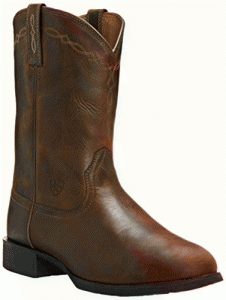## Bootstrap and Jacknife

### Bootstrap and Jackknife

Bootstrap and Jackknife algorithms don’t really give you something for nothing. They give you something you previously ignored.The jackknife is an algorithm for re-sampling from an existing sample to get estimates of the behavior of the single sample’s statistics. An example of the jackknife would be to omit the $$1^{st}, 2^{nd}, 3^{rd}$$ … observation from a sample of size $$n$$. Then compute the $$(n-1)$$ averages. The variance of these re-sampled averages is an estimate of the variance of the original sample mean.The bootstrap is a generalization of the jackknife that re-samples, with replacement, some number of times (say 1000), and computes the statistic of interest from these re-samples, thereby providing an estimate of the original sample’s variability. (e.g. The $$95^{th}$$ percentile is estimated by the $$950^{th}$$ observation of the ordered 1000 re-samples). The name, of course, comes from its apparent ability to pull itself up by its own bootstraps. (In Rudolph Erich Raspe’s tale, Baron Munchausen had fallen to the bottom of a deep lake and just as he was to succumb to his fate he thought to pull himself up by his own bootstraps.)

##### Cautions:

While this simple algorithm works quite well in many situations, it is termed a “naive” bootstrap because indiscriminate use can produce bogus results.  For example, a simple resampling of a time-series would ignore any auto-correlation and lead to spurious conclusions.  If the data are in $$x, y$$ pairs, then a naive resampling would be from the joint distribution of $$x$$ and $$y$$ when for regression purposes we need the conditional distribution of $$y$$, given $$x$$. And it isn’t magic: If what you’re looking for isn’t in the original sample, then it cannot appear in any of the resamples.

##### Reference:

Bradley Efron and Robert J. Tibshirani, An Introduction to the Bootstrap, Chapman and Hall, 1993